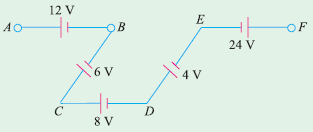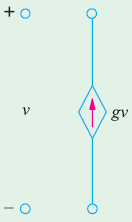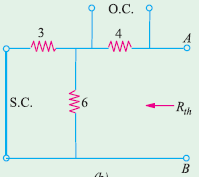# SSC JE Electrical Important MCQ Part 14

SSC JE Electrical Important MCQ PDF Part 14

1.Circuit shown in fig have two resistors of values 1 kΩ and 4 Ω are connected in series across a constant voltage supply of 100 V. A voltmeter having an internal resistance of 12 kΩ is connected across the 4 kΩ resistor.Actual voltage across 4kΩ resistor after the voltmeter is connectedA. 80 V

B. 20 V

C. 75 V

D. 65 V

(In Fig. voltmeter has been joined across the 4 kΩ resistor

The equivalent resistance between B and C = 4 × 12/16 = 3 kΩ

Voltage across B and C by voltage-divider rule= 100 × 3/(3 + 1) = 75 V)

2.In Fig. the values of VAF isA. − 6 V

B. 6 V

C. − 4 V

D. 4 V

(VAF stands for the potential of point A with respect to point F.So Starting from point F as we go to point A.

VAF = − 24 + 4 + 8 − 6 + 12 = − 6 V)

3.Circuit shown in fig. isA. Voltage-dependent voltage source

B. Current-dependent voltage source

C. Voltage-dependent current source

D. Current-dependent current source

4.The value of load resistance RL in the circuit of Fig. for maximum power isA. 6 Ω

B. 3 Ω

C. 8 Ω

D. 9 Ω

(We will remove the voltage and current sources as well as RL from terminals A and B in order to find RthFor maximum power RL=Rth=4 + 6 || 3 = 6 Ω)

5.Maxwell’s loop current method of solving electrical networks

A. uses branch currents

B. utilizes Kirchhoff’s voltage law

C. is confined to single-loop circuits

D. is a network reduction method

Answer  : B (This method consists of writing loop voltage equations by Kirchhoff’s voltage law in terms of unknown loop currents)

6.The algebraic sign of an IR drop is primarily dependent upon the

A. amount of current flowing through it

B. value of R

C. direction of current flow

D. battery connection

7.The current sensitivity of a meter is expressed in

A. ampere

B. ohm/ampere

C. ohm/volt

D. ampere/division

8.The basic meter movement can be converted into an ohmmeter by connecting a …..with it

A. high resistance in series

B. low resistance in parallel

C. battery in series

D. battery and a variable resistance in series

9.FETs have similar properties to

A. PNP transistors

B. NPN transistors

C. thermonic valves

D. unijunction transistors

10.The drain source voltage at which drain current becomes nearly constant is called

A. barrier voltage

B. breakdown voltage

C. pick-off voltage

D. pinch-off voltage

Answer  : D (Pinch off voltage is the drain to source voltage after which the drain to source current becomes almost constant and JFET enters into saturation region and is defined only when gate to source voltage is zero)

11.A magnetic amplifier can be used for the control of

A.Current

B. Voltage

C. Speed

D. All of these

Answer  : A (Current control is achieved by varying the magnetic flux in the cores using the appropriate DC current through the control winding)

12.Ward-Leonard controlled d.c. drives are generally used for

A. Light duty excavators

B. Medium duty excavators

C. Heavy duty excavators

D. All of these

Answer  : C (Ward-Leonard controlled d.c. drives  is commonly employed for elevators, hoists, and main drive in steel mills, as this method can give unlimited speed control in either direction. Since the generator voltage can be varied gradually from zero, no extra starting equipment is required to start up the main motor smoothly)

13.Spot welding process basically depends on

A. Ohmic resistance

B. Generation of heat

C. Application of forging pressure

D. Both Generation of heat and Application of forging pressure

14.Welding is not done directly from the supply mains because

A. It is customary to use welding machines

B. Its voltage is too high

C. Its voltage keeps fluctuating

D. It is impracticable to draw heavy currents

15.A large size alternator is protected against overloads by providing

A. Over current relays

B. Temperature sensitive relays

C. Thermal relays

D. None of these

Answer  : B (Overloading can causes overheating in the stator winding of the generator.The overheating is detected by temperature sensitive relays)

16.If the fault current is 2000 amps, the relay setting 50% and the C.T. ratio is 400/5, then the plug setting multiplier will be

A. 25 amps

B. 45 amps

C. 50 amps

D. 10 amps

(Given CT ratio=400/5

CT rated current is 5A

Relay setting is 50%, relay will operate for=5*50/100=2.5 A

Plug setting multiplier = Fault current/Relay setting*CT ratio
=2000/(2.5*400/5)
=10 A)

17.The normal practice to specify the making current of a circuit breaker is in terms of

A. r.m.s. value

B. peak value

C. average value

D. both r.m.s. and peak value

18.For reducing tower footing resistance it is better to use

A. chemical and ground rods only

B. chemical an counterpoise only

C. ground rod and counterpoise only

D. chemical, ground rods and counterpoise

19. Ferranti effect on long overhead lines is experienced when it is

B. on full load at unity p.f.

C. on full load at 0.8 p.f. lag

D. in all these cases

20.Insulation of the modern EHV lines is designed based on

A.Lighting voltage

B. Switching voltage

C. Corona

D. All of these